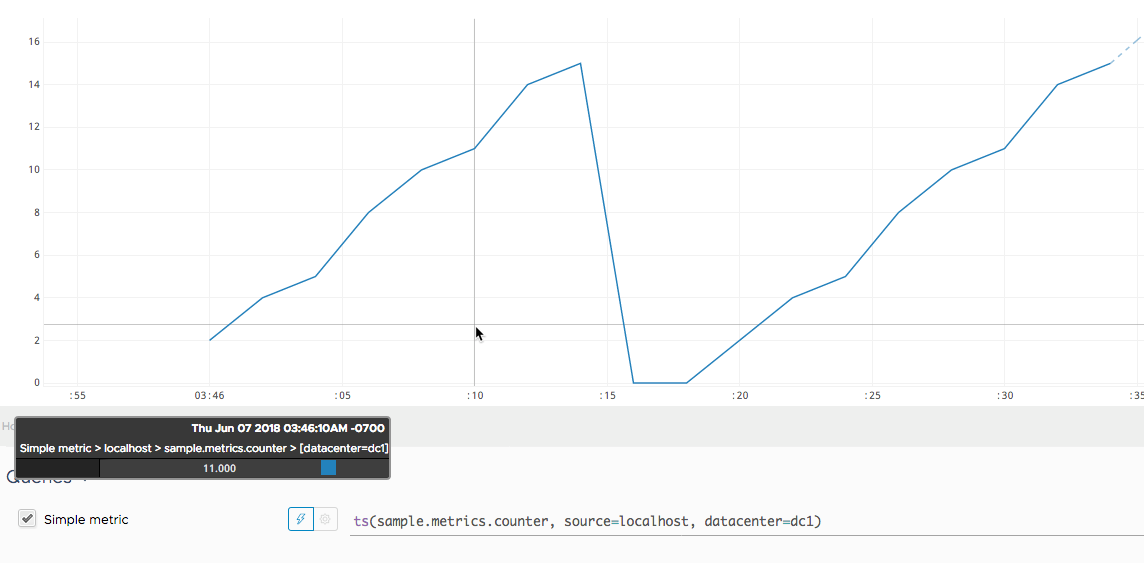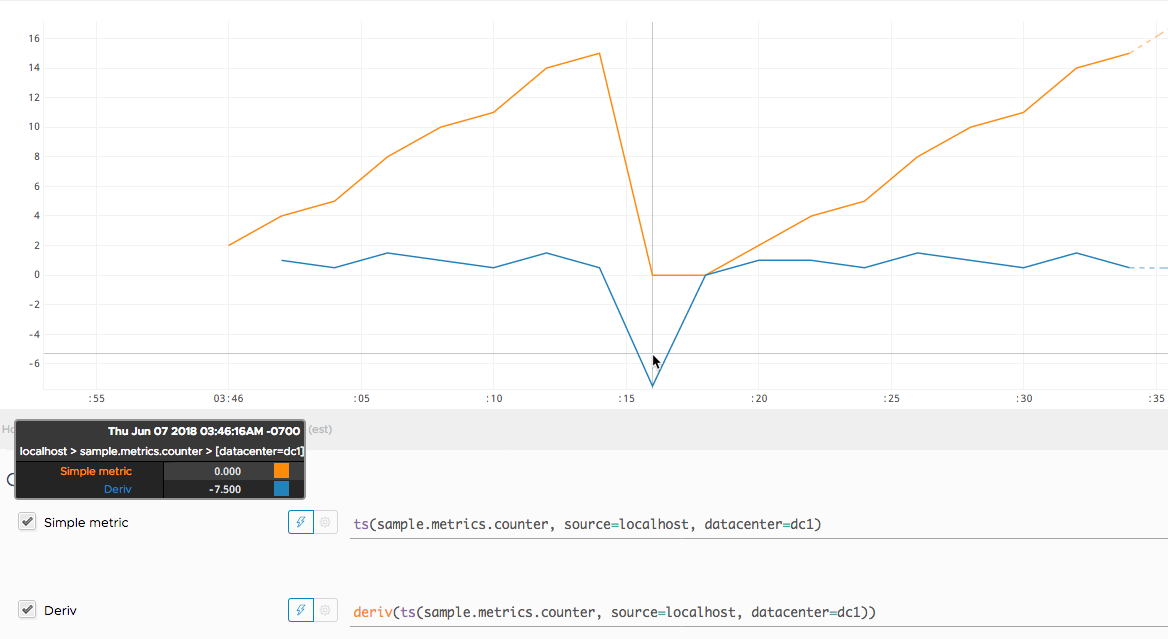Reference to the deriv() function

## Summary

``````deriv(<tsExpression>)
``````

Returns the per-second rate of change for each time series described by the tsExpression. The results can include both positive and negative rates of change. Use `rate()` if you want to see only positive rates of change.

## Parameters

ParameterDescription
tsExpression Expression that describes the time series to return rates of change for.

## Description

The `deriv()` standard time function returns the rate of change (per second) between adjacent data values in the time series described by the expression. `deriv()` returns a separate series of results for each time series described by the expression.

You can apply `deriv()` to any kind of metric. If you are primarily interested in the rates of change of counter metrics, however, you will get more useful results from `rate()`, which ignores negative rates of change.

### Rate of Change

`deriv()` finds the per-second rate of change between pairs of adjacent (successively reported) data values. The rate of change between a pair of data values is computed as follows:

1. Subtract the earlier data value from the later value.
2. Divide the difference by the number of seconds in the reporting interval.

For example, let’s say that a metric has a reporting interval of 30 seconds, and reports successive data values:

 Value Time 105,500 05:45:00pm 105,750 05:45:30pm

The `deriv()` function computes the rate of change between these data values using the following formula: `(105,750 - 105,500)/30`. The resulting positive value (8.333) is returned at 05:45:30pm, indicating that the metric increased by 8.333 per second between the two values.

Notice that the per-second rate of change between the same two data values would be quite different if the metric had reported them two minutes (120 seconds) apart: `(105,750 - 105,500)/120`. In this case, the returned per-second rate would be 2.083.

Now let’s say the above metric reports a later value that is less than the earlier value:

 Value Time 105,750 05:45:30pm 105,250 05:46:00pm

The `deriv()` function computes the rate of change between these data values using the following formula: `(105,250 - 105,750)/30`. The resulting negative value (-16.666) is returned at 05:46:00pm, indicating that the metric decreased by 16.666 per second between the two values. (In contrast, `rate()` simply produces a gap in this case, rather than reporting a negative rate of change.)

## Examples

Here’s a query that shows a sample metric that increments a counter. The reporting interval is 2 seconds, which means the counter increments every 2 seconds. We see the count climb from 3:46:00 to 3:46:16, when it resets to 0. The counter restarts at 3:46:20.Now we apply `deriv()` to our original query to find out how fast the counter grows per second. Notice:

• `deriv()` starts reporting at the counter’s second value.
• At 3:46:10, the rate of growth is .500, indicating the total has grown half a unit per second since the preceding value.
• A negative rate is reported when the counter falls to 0.